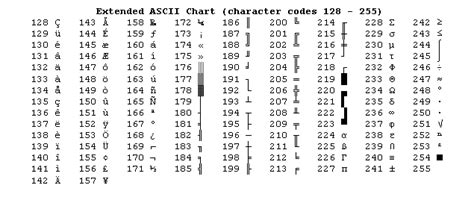# Neomatrix custom char?

Hello,

I’m using Neomatrix just for one line of text. It works fine.

How to add customs chars ? I would like to display bars for spectrum.

Thanks

Can you post a picture of your neomatrix?

Sorry I'm using the matrixText from Adafruit:

``````// Adafruit_NeoMatrix example for single NeoPixel Shield.
// Scrolls 'Howdy' across the matrix in a portrait (vertical) orientation.

#ifndef PSTR
#define PSTR // Make Arduino Due happy
#endif

#define PIN 6

// MATRIX DECLARATION:
// Parameter 1 = width of NeoPixel matrix
// Parameter 2 = height of matrix
// Parameter 3 = pin number (most are valid)
// Parameter 4 = matrix layout flags, add together as needed:
//   NEO_MATRIX_TOP, NEO_MATRIX_BOTTOM, NEO_MATRIX_LEFT, NEO_MATRIX_RIGHT:
//     Position of the FIRST LED in the matrix; pick two, e.g.
//     NEO_MATRIX_TOP + NEO_MATRIX_LEFT for the top-left corner.
//   NEO_MATRIX_ROWS, NEO_MATRIX_COLUMNS: LEDs are arranged in horizontal
//     rows or in vertical columns, respectively; pick one or the other.
//   NEO_MATRIX_PROGRESSIVE, NEO_MATRIX_ZIGZAG: all rows/columns proceed
//     in the same order, or alternate lines reverse direction; pick one.
//   See example below for these values in action.
// Parameter 5 = pixel type flags, add together as needed:
//   NEO_KHZ800  800 KHz bitstream (most NeoPixel products w/WS2812 LEDs)
//   NEO_KHZ400  400 KHz (classic 'v1' (not v2) FLORA pixels, WS2811 drivers)
//   NEO_GRB     Pixels are wired for GRB bitstream (most NeoPixel products)
//   NEO_RGB     Pixels are wired for RGB bitstream (v1 FLORA pixels, not v2)

// Example for NeoPixel Shield.  In this application we'd like to use it
// as a 5x8 tall matrix, with the USB port positioned at the top of the
// Arduino.  When held that way, the first pixel is at the top right, and
// lines are arranged in columns, progressive order.  The shield uses
// 800 KHz (v2) pixels that expect GRB color data.
NEO_MATRIX_TOP     + NEO_MATRIX_RIGHT +
NEO_MATRIX_COLUMNS + NEO_MATRIX_PROGRESSIVE,
NEO_GRB            + NEO_KHZ800);

const uint16_t colors[] = {
matrix.Color(255, 0, 0), matrix.Color(0, 255, 0), matrix.Color(0, 0, 255) };

void setup() {
matrix.begin();
matrix.setTextWrap(false);
matrix.setBrightness(40);
matrix.setTextColor(colors);
}

int x    = matrix.width();
int pass = 0;

void loop() {
matrix.fillScreen(0);
matrix.setCursor(x, 0);
matrix.print(F("Howdy"));
if(--x < -36) {
x = matrix.width();
if(++pass >= 3) pass = 0;
matrix.setTextColor(colors[pass]);
}
matrix.show();
delay(100);
}
``````

Thanks

No idea, how to display custom char ?

ThanksThis is the extended ASCII table. It has some special characters that might be useful.
You can create them by setting a character variable to the number value of the character, cast as a character.
Try it and see what you get.
Your next response should have a code example if you are having issues, as well as the previously requested picture of your neomatrix.

Hi,

I'm using 2 8*8 matrix

With this code:

`````` // Adafruit_NeoMatrix example for single NeoPixel Shield.
// Scrolls 'Howdy' across the matrix in a portrait (vertical) orientation.

#ifndef PSTR
#define PSTR // Make Arduino Due happy
#endif

#define PIN 4  //MUST BE A PWM PIN

// MATRIX DECLARATION:
// Parameter 1 = width of NeoPixel matrix
// Parameter 2 = height of matrix
// Parameter 3 = pin number (most are valid)
// Parameter 4 = matrix layout flags, add together as needed:
//   NEO_MATRIX_TOP, NEO_MATRIX_BOTTOM, NEO_MATRIX_LEFT, NEO_MATRIX_RIGHT:
//     Position of the FIRST LED in the matrix; pick two, e.g.
//     NEO_MATRIX_TOP + NEO_MATRIX_LEFT for the top-left corner.
//   NEO_MATRIX_ROWS, NEO_MATRIX_COLUMNS: LEDs are arranged in horizontal
//     rows or in vertical columns, respectively; pick one or the other.
//   NEO_MATRIX_PROGRESSIVE, NEO_MATRIX_ZIGZAG: all rows/columns proceed
//     in the same order, or alternate lines reverse direction; pick one.
//   See example below for these values in action.
// Parameter 5 = pixel type flags, add together as needed:
//   NEO_KHZ800  800 KHz bitstream (most NeoPixel products w/WS2812 LEDs)
//   NEO_KHZ400  400 KHz (classic 'v1' (not v2) FLORA pixels, WS2811 drivers)
//   NEO_GRB     Pixels are wired for GRB bitstream (most NeoPixel products)
//   NEO_RGB     Pixels are wired for RGB bitstream (v1 FLORA pixels, not v2)

// Example for NeoPixel Shield.  In this application we'd like to use it
// as a 5x8 tall matrix, with the USB port positioned at the top of the
// Arduino.  When held that way, the first pixel is at the top right, and
// lines are arranged in columns, progressive order.  The shield uses
// 800 KHz (v2) pixels that expect GRB color data.
NEO_MATRIX_TOP     + NEO_MATRIX_RIGHT +
NEO_MATRIX_COLUMNS + NEO_MATRIX_PROGRESSIVE,
NEO_GRB            + NEO_KHZ800);

const uint16_t colors[] = {
matrix.Color(255, 0, 0), matrix.Color(0, 255, 0), matrix.Color(0, 0, 255) };

void setup() {
matrix.begin();
matrix.setTextWrap(false);
matrix.setBrightness(20);
matrix.setTextColor(colors);
}

int x    = matrix.width();
int pass = 0;

void loop() {
matrix.fillScreen(0);
matrix.setCursor(0, 0);
matrix.print(String(char(31)) + String(char(218)));
/* if(--x < -36) {
x = matrix.width();
if(++pass >= 3) pass = 0;
matrix.setTextColor(colors[pass]);
}*/
matrix.show();
delay(100);
}
``````

I tried extended ASCII table. It works. Not are 100% the same as the standard table but you can have special chars. But I haven't found what I'm looking for.

I would like bar progression. From one bar (5x1) on the bottom, 2 bars (5x2), 3 bars (5x3),... to (5x8) . For this one char 218 is perfect.

Thanks

1 Like

This is an awesome library. Since the extended ascii isn't exactly what he's looking for this would be my next recommendation.
Also, since just rectangles are needed it might be just as fast to write a function
rectangle(x, y, width, height)

Thanks. It seems easier to modify the existing files (replace extended chars by mines). But I don't know what is the font used by default. Nothing is selected in the code?
Thanks

Where is the "default" font files hidden ?

Thanks

How is that easier?
Have you tried just drawing a box?

I need to include custom chars together with normal chars !

Where is the "default" font files hidden ?